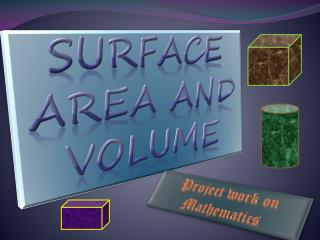# Surface area and volume - PowerPoint PPT PresentationDownload PresentationSurface area and volume

Surface area and volumeDownload Presentation## Surface area and volume

- - - - - - - - - - - - - - - - - - - - - - - - - - - E N D - - - - - - - - - - - - - - - - - - - - - - - - - - -
##### Presentation Transcript

1. Surface area and volume Project work on Mathematics

2. CUBE :- A solid three-dimensional geometric figure bounded by six planes; each of the six sides or faces of a cube is a a2 a3 a1

3. a FINDING THE SURFACE AREA OF CUBE. a a a a a a a a a a a

4. FINDING LATERAL SURFACE AREA OF CUBE:-

5. CUBOID :- A three-dimensional geometric figure formed of six rectangular plane faces, each set at right angles to the four sides adjacent to it. h b l

6. l b b l h FINDING TOTAL SURFACE AREA OF CUBOID:- h h h l b b l

7. FINDING LATERAL SURFACE AREA OF CUBOID :-

8. r h CYLINDER:- Cylinder consists of two circular bases of equal area in parallel planes, that are connected by a lateral surface that intersects the boundaries of the bases.

9. FINDING CURVED SURFACE AREA OF CYLINDER:- r h

10. l h r CONE :- A surface generated by a straight line that moves along a closed curve while always passing through a fixed point.

11. FINDING CURVED SURFACE AREA OF CONE :- l h r

12. r SPHERE :- Surface of which every point is the same distance from a fixed point known as the centre. From every aspect it has the same appearance. A sphere may be described by the rotation of a semicircle or circle, about its diameter.

13. r Hemisphere :- ‘Hemi’ also means ‘half’. So When Sphere is Divided into two equal parts Each equal part is called Hemisphere